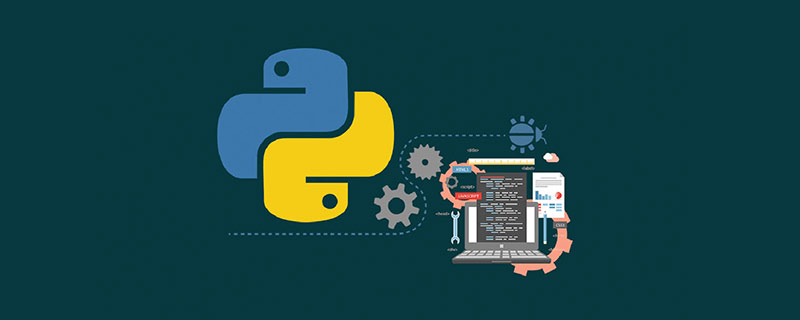# python什么是反序列化？```>>> import pickle
>>> d=[1,2,3,4]
>>> pickle.dumps(d)
b'\x80\x03]q\x00(K\x01K\x02K\x03K\x04e.'```

dumps将所传入的变量的值序列化为一个bytes，然后，就可以将这个bytes写入磁盘或者进行传输。

```f=open('file_test','wb')
>>> d=[1,2,3,4]
>>> pickle.dump(d,f)
>>> f.close()
>>> f=opem('file_test','rb')
f=open('file_test','rb')
b'\x80\x03]q\x00(K\x01K\x02K\x03K\x04e.'```

```>>> d=[1,2,3,4]
>>> r=pickle.dumps(d)
>>> print(r)
b'\x80\x03]q\x00(K\x01K\x02K\x03K\x04e.'
[1, 2, 3, 4]```
```>>> d=[1,2,3,4]
>>> f=open('file_test','wb')
>>> pickle.dump(d,f)
>>> f.close()
>>> f=open('file_test','rb')